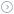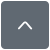如果没坏，就不要修理，调节固定增益差分放大器的增益

|G0 =G_attn×噪声增益噪声增益= 1/β–β–=G_attn/G0β–=G_attn/G0 (1)

 βc =G_attn/G1βc =β––β+β+ =G_attn(1/G0–1/G1) (2)

β+的量正好是输出信号返回至运算放大器的同相输入端的一部分。记住，反馈会通过β+路径至基准引脚，反馈信号会通过两个电阻的分压器（见图3），这两个分压电阻阻值必须要通过计算才能实现正确的β+。

 让(1/G_attn)–1 =α (3)

 Vth×α/(α+ 1) =VA_in+因为VA_in+/VOUT =β+其中β+ =G_attn(1/G0–1/G1)R4/(R3 + R4)) = (1/α)×(1/G0–1/G1) (4)R3和R4可以使用公式6计算得出：让R3 + R4 = 2000，R4 = 30，R3 = 1970，R5 = 1773 (8)

 让R3 = 2050，R4 = (32.4 || 866)，R5 = (1910 || 54900) (9)『本文转载自网络,版权归原作者所有,如有侵权请联系删除』热门文章 更多